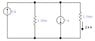# Parallel circuit problem

• Engineering
saulwizard1

## Homework Statement

Solve the next circuit to find the voltages

I=V/R
GT=(1/R1)+(1/Rn)
V=V1=V2=V3

## The Attempt at a Solution

My background is that I know that in a parallel circuit, the voltage for each component is the same and electric current it's equal to the sum of the electric currents of each one of the resistances by separate. My first and only attempt was to multiply the 5A by 3 ohms.

#### Attachments

•15-5.jpg
5.8 KB · Views: 377
Last edited:

Mentor
Your problem statement is not clear or complete. What do you mean by "Solve the next circuit"? Solve for what? Please make an effort to provide clear problem statements that can be readily understood by the Homework Helpers.

What was your motivation for multiplying 5A by 3 Ohms? What about the 1A source and the other resistor?

Hint: You are given a current through one of the resistors. Does that give you any useful voltage information?

•amrmohammed
saulwizard1
Your problem statement is not clear or complete. What do you mean by "Solve the next circuit"? Solve for what? Please make an effort to provide clear problem statements that can be readily understood by the Homework Helpers.

What was your motivation for multiplying 5A by 3 Ohms? What about the 1A source and the other resistor?

Hint: You are given a current through one of the resistors. Does that give you any useful voltage information?

According what I understand, the 2.4A would help me to get the voltage using Voltage=(current)(resistance), or in what way can it help me?

Mentor
According what I understand, the 2.4A would help me to get the voltage using Voltage=(current)(resistance), or in what way can it help me?

•amrmohammed
saulwizard1
•